# Errors, Theory of

## Errors, Theory of

the branch of mathematical statistics concerned with the analysis of measurement errors and with the refinement of the numerical values of approximately measured quantities. Repeated measurements of a constant quantity generally yield different results, since each measurement contains some error. There are three basic kinds of errors: systematic errors, blunders, and random errors. Systematic errors consistently either exaggerate or understate the results of measurements and have specific causes (the measuring instruments are improperly set up, an environmental factor) that systematically influence and alter the results of the measurements. Such errors are estimated by methods that lie beyond the purview of mathematical statistics. Errors in counting and the incorrect reading of instruments are among the causes of blunders. The results of measurements involving blunders differ markedly from other measurement results and therefore are often easily noticed. Random errors stem from various random factors that act in an unforeseen manner during each individual measurement—sometimes decreasing and sometimes increasing the results.

The theory of errors deals only with blunders and random errors. Its basic problems are the ascertainment of the laws of distribution of random errors; establishment, based on measurement results, of estimates of the unknown quantities being measured; determination of the errors in the estimates; and elimination of blunders.

Let the values x1, x2, …, xn be obtained from n independent, equally accurate measurements of the quantity a. The differences

δ1 = x1a, …, δn = xna

are called the true errors. In terms of the probabilistic theory of errors, the δi are interpreted as random quantities; the independence of the measurements is taken to mean the mutual independence of the random quantities δ1,…, δn. The equal accuracy of the measurements is interpreted in the broad sense as identical distribution; that is, the true errors of equally accurate measurements are identically distributed random quantities. The mathematical expectation of the random errors b = Eδ1 = … = Eδn is called the systematic error, and the differences δ1b, …, δnb are called random errors. Thus, the absence of a systematic error means that b = 0 and δ1, …, δn are random errors. The quantity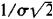, where σ is the root-mean-square deviation, is called the index of precision. When a systematic error is present, the index of precision is expressed by the ratio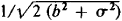. In the narrow sense, equal accuracy of measurements means that the index of precision is identical for all measurement results. The presence of blunders indicates that equal accuracy in both the narrow and the broad senses has been violated for some individual measurements. The arithmetic mean of the results of the measurements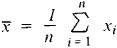is usually selected as an estimate of the unknown quantity a, and the differences Δ1 = x1, …, Δn = xn are called the apparent errors. The selection of as an estimate for α is based on the law of large numbers: when the number n of equally accurate measurements lacking a systematic error is sufficiently large, the estimate x differs arbitrarily little from the unknown quantity α with a probability arbitrarily close to unity. The estimate x lacks systematic error—estimates with this property are said to be unbiased. The deviation of the estimate is

Dx̄ = E(x̄ – α)2 = σ2n

Experience shows that the random errors σi often conform to distributions close to the normal distribution; the reasons for this are given by the limit theorems of probability theory. In this case, the quantity x has a distribution that differs little from a normal distribution with a mathematical expectation α and deviation σ2/n. If the distributions of σi are precisely normal, then the deviation of any other unbiased estimate for α, such as a median, is at least Dx. But this property does not hold if the distribution of σi is non-normal.

If the deviation σ2 of the individual measurements is not known in advance, it can be estimated by the quantity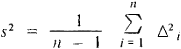Es2 = σ2; that is, s2 is an unbiased estimate for σ2. If the random errors δi have a normal distribution, the relation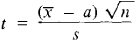obeys Student’s distribution with n – 1 degrees of freedom. This fact can be used to estimate the error of the approximate equality a.

On the same assumptions, the quantity (n – 1) s2/σ2 has the chi-squared distribution with n – 1 degrees of freedon. This fact permits estimation of the error of the approximate equality σs. It can be shown that the relative error ǀsσǀ/s will not exceed the number q with the probability

ω = F(z2, n – 1) – F(z1 n – 1)

where F (z, n – 1) is a function of the chi-squared distribution and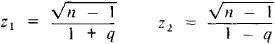### REFERENCES

Linnik, Iu. V. Metod naimen’shikh kvadratov i osnovy matematiko-statisticheskoi teorii obrabotki nabliudenii. 2nd ed. Moscow, 1962.
Bol’shev, L. N., and N. V. Smirnov. Tablitsy matematicheskoi statistiki, 2nd ed. Moscow, 1968.

L. N. BOL’SHEV

Site: Follow: Share:
Open / Close